# Python enumerations

## Definition of enumerations in Python

Enumeration in Python is simply a collection of symbolic names that are bound to unique and constant values. An enumeration in Python can be iterated. The example to show how enumeration in Python can be iterated is shown later on in this page.

The "enum" module is used to implement enumeration in Python. And classes are used to create enumerations, like shown in the code block given below:

```from enum import Enum
class OrderCount(Enum):
LAPTOP = 3
MOBILE = 6
TV = 2```

Note: In the above example, I've written all the enum members in UPPERCASE letters because enumeration is used to represent constants. And it is better practice to write any variable that holds constant values in uppercase. Otherwise, it is up to you whether to use uppercase, lowercase, or a combination of the two.

In the above example of enumeration:

• The class named "OrderCount" is an enumeration (or enum in short).
• The attributes OrderCount.LAPTOP, OrderCount.MOBILE, etc. are enumeration members that are functional constants.
• As you can see, enumerations are made up of a name and a value pair.
• The name of OrderCount.LAPTOP is LAPTOP, and the value of OrderCount.LAPTOP is 3.

## Enumeration Methods and Properties

Now we've got to learn these 5 things that are most widely used in Python enumerations. I kept the above short block of code in mind while evaluating these things with the help of an example to give you a clear idea of the subject.

1. repr() returns the official string representation of the value that is being passed. For example, "print(repr(OrderCount.LAPTOP))" produces "<OrderCount.LAPTOP: 3>" on the output console from the previous example. That is, enumeration members have human-readable string representations. But using "repr()," we will be able to fetch the official or the detailed version of the string.
2. type() returns the enumeration's name that the enumeration member belongs to. For example, "print(type(OrderCount.LAPTOP))" produces "<enum 'OrderCount'>" on the output console. That shows the "OrderCount.LAPTOP" enum member belongs to the "OrderCount" enum.
3. isinstance() returns true if the specified object (the first argument) is an instance of the class (the second argument). Otherwise, it returns false. For example, "print(isinstance(OrderCount.LAPTOP, OrderCount))" produces true on the output console since "OrderCount.LAPTOP" (the first argument) is an instance of the class named "OrderCount" (the second argument).
4. name: In Python, "enum" members have a property that contains the name of the item. For example, "print(OrderCount.LAPTOP.name)" produces "LAPTOP" on the output. That is the name of the "enum" item.
5. value: In Python, "enum" members also have a property that contains the value of the item. For example, "print(OrderCount.LAPTOP.value)" produces 3 on the output.

### Implementation of enumeration methods and properties as an example

The program given below uses repr(), type(), isinstance(), name, and value in one single program. Let's have a look at the program given below and understand it in a practical way after seeing its sample output:

```from enum import Enum

class OrderCount(Enum):
LAPTOP = 3
MOBILE = 6
TV = 2

print(repr(OrderCount.LAPTOP))
print(type(OrderCount.LAPTOP))
print(isinstance(OrderCount.LAPTOP, OrderCount))
print(OrderCount.LAPTOP.name)
print(OrderCount.LAPTOP.value)```

Here is its sample output: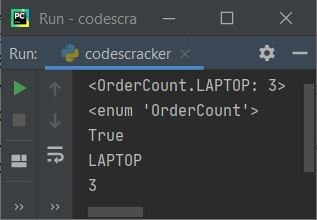## Enumeration implementation example

Now let's take a look at the program given below that demonstrates enumeration in a practical way. This is basically a modified version of the previous program. Since this program includes a description of each output:

```from enum import Enum

class OrderCount(Enum):
LAPTOP = 3
MOBILE = 6
TV = 2

print("The string representation of enum member \"OrderCount.MOBILE\" is:", OrderCount.MOBILE)
print("The \"repr\" representation of enum member \"OrderCount.MOBILE\" is:", repr(OrderCount.MOBILE))
print("The name of enum member \"OrderCount.MOBILE\" is:", OrderCount.MOBILE.name)
print("The value of enum member \"OrderCount.MOBILE\" is:", OrderCount.MOBILE.value)```

The snapshot given below shows its sample output: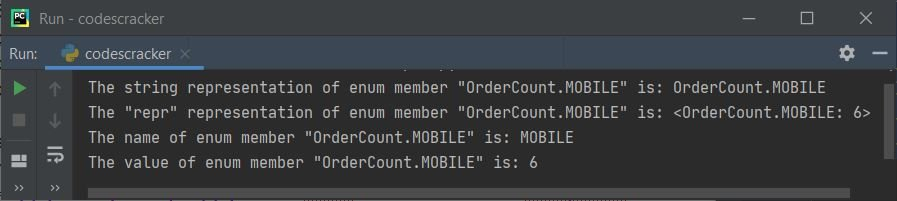### Print all enumeration values using "enum" iteration

This example is created in such a way that it will create an enumeration and then print all the values of the enumeration:

```from enum import Enum

class OrderCount(Enum):
LAPTOP = 3
MOBILE = 6
TV = 2

print("All enum values are:")
for val in OrderCount:
print(val.value)```

This program produces the output that looks like: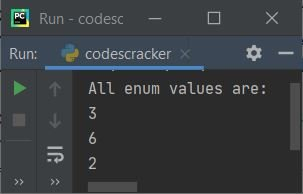### Print all the enumeration items in name-value pairs

Basically, this is a modified and improved version of the previous program. This program prints all enumeration items' names along with their values in an organized way:

```from enum import Enum

class OrderCount(Enum):
LAPTOP = 3
MOBILE = 6
TABLET = 2

print("All enum Names - values are:")
print("\nName\t\tValue")
print("-----------------")
for val in OrderCount:
print(val.name, "\t\t", val.value)```

And the snapshot given below shows the sample output produced by the above Python program: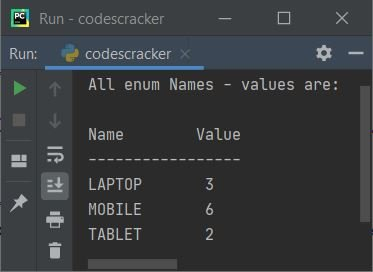## Enumeration members are hashable

Since enumeration members in Python are hashable, we can use them in dictionaries or sets. If any object never changes its value during its lifetime, then the object is called a hashable object. In other words, hashability enables an object to be usable as a dictionary key and a set member.

The example program given below illustrates that all the things said here to support enum members are hashable.

```from enum import Enum

class Product(Enum):
MOBILE = 3
LAPTOP = 6
TABLET = 5

product_dict = {}
product_dict[Product.MOBILE] = 'white'
product_dict[Product.LAPTOP] = 'black'

if product_dict == {Product.MOBILE: 'white', Product.LAPTOP: 'black'}:
print("Enum members are hashable")
else:
print("Enum members are not hashable")```

The image below shows an example of the output this Python program produces: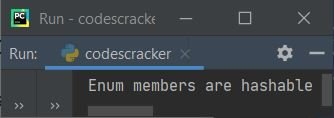Python Online Test

« Previous Tutorial Next Tutorial »